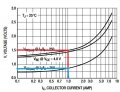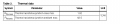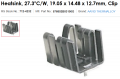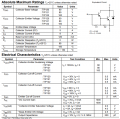# tip120 12v system

#### jimsjump

Joined Nov 4, 2020
14
Hi, I have been building a circuit that utilises a 555 timer to switch a tip120. The whole system is 12v.
is it possible to safely attach 12v to the base of this transistor to allow 12v to travel through the collector and emitter?
it has to run a load of 1amp.
I so what would be the current limiting resistor to use on the base?
At the moment i am using 470Ω and it seems to be working fine but not sure for how long..
I have looked at all the calculations and am still getting my head around it all.

#### atferrari

Joined Jan 6, 2004
4,699
Show the schematic you are trying to describe.

#### jimsjump

Joined Nov 4, 2020
14

#### LesJones

Joined Jan 8, 2017
4,013
470 ohms should be fine. This will give a base emitter current of (12 - 1.4)/470 = 10.6 /470 = 0.023 amps = 23 mA
(The 1.4 value is due to the two base emitter junctions voltage drops due to the fact that the TIP120 is a darlington transistor.)
23 mA is well below the maximum base emitter current rating of 120 mA and is enough to drive the transistor into saturation with 1 amp collector current.

Les.

•Irving

#### jimsjump

Joined Nov 4, 2020
14
470 ohms should be fine. This will give a base emitter current of (12 - 1.4)/470 = 10.6 /470 = 0.023 amps = 23 mA
(The 1.4 value is due to the two base emitter junctions voltage drops due to the fact that the TIP120 is a darlington transistor.)
23 mA is well below the maximum base emitter current rating of 120 mA and is enough to drive the transistor into saturation with 1 amp collector current.

Les.
Thank you, hopefully i can now start to get a better understanding of the calculations.

#### crutschow

Joined Mar 14, 2008
32,849
The data sheet for the TIP120 (below) shows that for minimum saturation (ON) voltage, the base current should be about 1/250 times the collector current (forced Beta of 250).
So for a collector current of 1A you need at least 1/250 = 4mA of base current.
You could thus increase the base resistor to about 2.5kΩ if you like, and still fully turn on the transistor.•Irving

#### Irving

Joined Jan 30, 2016
3,496
Following on from @LesJones (edit: and now @crutschow 's) excellent reply, here's how to read the datasheet (attached) to do all the calculations you need:

Starting with the 'On' voltages chart in Fig11 (LH chart for NPN TIP120) we use an arbitrary transistor gain (base current Ib to collector current Ic) of 250 (noting minimum guaranteed gain is 1000, so giving significant safety margin):Read off (red line) for Ic = 1A (and therefore Ib = 1/250 = 4mA) that the voltage between base and emitter, Vbe(sat) is 1.5v (close to LJ's 'rule of thumb' of 1.4v). Therefore the required maximum base resistor value is (Vsupply - Vbe)/Ib = (12 - 1.5)/0.004 = 2625ohm

Read off (blue line) for Ic = 1A that the voltage between collector and emitter, Vce(sat), is approx 0.7v. Therefore the bulb will see (12 - 0.7) = 11.3V, which as near 12v as makes no difference for a bulb (but for other devices, such as a relay or a motor, this figure could have an impact).

The current base resistor is 470ohm. While is this a not a bad choice, its not a great choice. Why? Well, 470ohm across 10.5v will dissipate V^2/R W of heat, or (12 - 1.5)^2/470 = 0.24W so that needs to be a 0.5W or bigger resistor and could get fairly warm. A bigger resistor, 1.5k or 2.2k would be better, generating only 0.075W or 0.05W and allowing use of a standard 0.25W resistor, as well as being more efficient.

Why else do we need to know Vce(sat)? Well the transistor is dropping 0.7v at a current of 1A and therefore loosing Vce * Ic Watts of power, ie 0.7 * 1 = 0.7W as heat. It also looses Vbe(sat) * Ib W ie 1.5 * 0.023 = 0.035W, for a total of 0.735W. If we look elsewhere in the datasheet we find:The second parameter tells us that in free air for every Watt of heat generated the transistor's junction temperature rises 62.5degC above ambient. So in this case the junction temperature Tj will be 62.5 * 0.735 + 25 = 70.9degC and the case itself, using the first parameter, will be Tc = Tj - 1.92 * power loss =70.9 - 1.92 * 0.735 = 69.5degC, which is rather hot to the touch. I'd use a small clip-on heatsink like this one, which will reduce the junction temperature to 55degC and surface temp to 52degC. It will also allow you to increase collector current to at least 3A safely should you need to.#### Attachments

• 288.8 KB Views: 0
Last edited:

#### crutschow

Joined Mar 14, 2008
32,849
Following on from @LesJones excellent reply, here's how to read the datasheet (attached) to do all the calculations you need:
That graph is for the typical operation of the transistor (which the one you buy may not be).
As noted in the data sheet clip below, the minimum current gain of 1000 is for a Vce of 3V, not saturation.
So for operation as a switch, you should use a beta of 250, as I stated in my previous post.•Irving

#### Audioguru again

Joined Oct 21, 2019
6,151
Do you really have -12V or is it actually 0V?
For a load of only 1A and a total supply of only 12V then why use a darlington that reduces the voltage to the load by 1.2V max and heat the darlington? Use a normal TIP41 transistor instead.

The TIP41 has a max saturation voltage loss of only 0.15V max when it conducts 1A and has a base current of 100mA which the 555 can easily produce. The heating in the TIP41 will be only 0.24W max and will be slightly warm. The base resistor will be (12V - 0.9V - 1.6V)/100mA=95 ohms (use 100 ohms) and will heat with 0.9W so use a 2W resistor.

#### Delta Prime

Joined Nov 15, 2019
1,311

#### BobaMosfet

Joined Jul 1, 2009
2,081
Hi, I have been building a circuit that utilises a 555 timer to switch a tip120. The whole system is 12v.
is it possible to safely attach 12v to the base of this transistor to allow 12v to travel through the collector and emitter?
it has to run a load of 1amp.
I so what would be the current limiting resistor to use on the base?
At the moment i am using 470Ω and it seems to be working fine but not sure for how long..
I have looked at all the calculations and am still getting my head around it all.
From the datasheet:Max Vebo = 5VDC.

#### Attachments

• 250.3 KB Views: 2

#### Irving

Joined Jan 30, 2016
3,496
That graph is for the typical operation of the transistor (which the one you buy may not be).
As noted in the data sheet clip below, the minimum current gain of 1000 is for a Vce of 3V, not saturation.
So for operation as a switch, you should use a beta of 250, as I stated in my previous post.
Agreed, that's why I also used a beta of 250, and wrote:
... use an arbitrary transistor gain (base current Ib to collector current Ic) of 250 (noting minimum guaranteed gain is 1000, so giving significant safety margin)
Yes, the graphs are typical but Vbe(sat) and Vce(sat) v Ic are given at that lower beta of 250 and at a low Ic of 1A is pretty constant across devices at room temp - I've used them extensively for a couple of commercial projects and you'd be very unlucky to find a significant outlier until you push them.

#### Irving

Joined Jan 30, 2016
3,496
@BobaMosfet Not sure what you were trying to say here?
Max Vebo = 5VDC.
Vebo is the reverse voltage ie emitter +ve wrt base,and is less than 12v but not sure how that's relevant to the TS's question?

#### Irving

Joined Jan 30, 2016
3,496
@jimsjump
While what you're proposing works, its not optimal. As suggested by @Audioguru again there are better transistors but ultimately these days I'd suggest using a MOSFET.

An IRF520 for instance would give a Vds saturation voltage of <0.3v at 1A and run 30degC cooler...

#### Audioguru again

Joined Oct 21, 2019
6,151
Yes, use a Mosfet instead. It and its gate resistor also will not produce any heat, and the load will get most of the 12V.

•BobaMosfet

#### BobaMosfet

Joined Jul 1, 2009
2,081
@BobaMosfet Not sure what you were trying to say here?

Vebo is the reverse voltage ie emitter +ve wrt base,and is less than 12v but not sure how that's relevant to the TS's question?
I'm sorry, I was actually thinking Vbe(on) = 2.5VDC (max). Looking at TP's original schematic I = E/R, which is 12-2.5 / 470 - 20mA. Some people had just assumed Vbe(on) of 1.4 because it's a Darlington, but it's safer to use the datasheet value of 2.5.

#### Delta Prime

Joined Nov 15, 2019
1,311
I have looked at all the calculations and am still getting my head around it all.
Stay the course learn and have fun that's what it's all about besides who doesn't like Ferraris you could use mosfets another day and believe me you will